# Weighted Average Cost of Capital (WACC)

The weighted average cost of capital (WACC) is one of the key inputs in discounted cash flow (DCF) analysis and is frequently the topic of technical investment banking interviews. The WACC is the rate at which a company’s future cash flows need to be discounted to arrive at a present value for the business. It reflects the perceived riskiness of the cash flows. Put simply, if the value of a company equals the present value of its future cash flows, WACC is the rate we use to discount those future cash flows to the present.

## The WACC formula

Below we present the WACC formula. To understand the intuition behind this formula and how to arrive at these calculations, read on.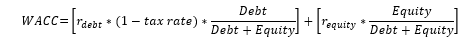Where:

## Cost of capital basics

Before getting into the specifics of calculating WACC, let’s understand the basics of why we need to discount future cash flows in the first place. We’ll start with a simple example:

Suppose I promise to give you \$1,000 next year in exchange for money upfront. What’s the most you would be willing to pay me for that today? Would you be willing to pay me \$500? What about \$800?  Your decision depends on the risk you perceive of receiving the \$1,000 cash flow next year.

• Low risk = low return: If you feel there is little to no risk of not getting paid, you would quantify your opportunity cost as low. You’d be willing to pay more, and thus receive a lower return.
• High risk = high return: If you feel the chance of actually collecting the \$1,000 is very low, you may not be willing to part with much money today. In other words, you’d discount at a high rate.

It should be easy from this example to see how higher perceived risk correlates to a higher required return and vice versa. The challenge is how to quantify the risk. The WACC formula is simply a method that attempts to do that.

We can also think of this as a cost of capital from the perspective of the entity raising the capital. (In our simple example, that entity is me, but in practice it would be a company.) If I promise you \$1,000 next year in exchange for money now, the higher the risk you perceive equates to a higher cost of capital for me.

Here’s an easy way to see this: Imagine you decide there’s a high risk of me not paying you \$1000 in the future, so you’re only willing to give me \$500 today. For me, that amounts to a 100% interest rate (\$500 principal return + \$500 in interest).

It should be clear by now that raising capital (both debt and equity) comes with a cost to the company raising the capital: The cost of debt is the interest the company must pay. The cost of equity is dilution of ownership.

While our simple example resembles debt (with a fixed and clear repayment), the same concept applies to equity.  The equity investor will require a higher return (via dividends or via a lower valuation), which leads to a higher cost of equity capital to the company because they have to pay the higher dividends or accept a lower valuation, which means higher dilution of existing shareholders.

It should be clear by now that raising capital (both debt and equity) comes with a cost to the company raising the capital: The cost of debt is the interest the company must pay. The cost of equity is dilution of ownership. From the lender and equity investor perspective, the higher the perceived risks, the higher the returns they will expect, and drive the cost of capital up. It’s two sides of the same coin.

### The risk-free rate

Let’s get back to our simplified example, in which I promise to give you a \$1,000 next year, and you must decide how much to give me today. Even if you perceive no risk, you will most likely still give me less than \$1,000 simply because you prefer money in hand. The rate you will charge, even if you estimated no risk, is called the risk-free rate. When investors purchase U.S. treasuries, it’s essentially risk free — the government can print money, so the risk of default is zero (or close to it). The return on risk-free securities is currently around 2.5%. Because you can invest in risk-free U.S. treasuries at 2.5%, you would be crazy to give me any more than \$1,000/1.025 = \$975.61.

As we’ll see, it’s often helpful to think of cost of debt and cost of equity as starting from a baseline of the risk-free rate + a premium above the risk-free rate that reflects the risks of the investment.Step-by-Step Online Course

#### Everything You Need To Master Financial Modeling

Enroll in The Premium Package: Learn Financial Statement Modeling, DCF, M&A, LBO and Comps. The same training program used at top investment banks.

## Capital structure

Now that we’ve covered the high-level stuff, let’s dig into the WACC formula. Recall the WACC formula from earlier:Notice there are two components of the WACC formula above: A cost of debt (rdebt) and a cost of equity (requity), both multiplied by the proportion of the company’s debt and equity capital, respectively.

### Capital structure — a company’s debt and equity mix

Lender risk is usually lower than equity investor risk because debt payments are fixed and predictable, and equity investors can only be paid after lenders are paid. Also, companies are typically under no obligation to make equity payments (like the issuance of dividends) within a certain time window. They can choose to delay payments until some event in the future such as an acquisition. This makes cash flows even less predictable (read: risky) for equity investors.

Because the cost of debt and cost of equity that a company faces are different, the WACC has to account for how much debt vs equity a company has, and to allocate the respective risks according to the debt and equity capital weights appropriately. In other words, the WACC is a blend of a company’s equity and debt cost of capital based on the company’s debt and equity capital ratio. As such, the first step in calculating WACC is to estimate the debt-to-equity mix (capital structure).

#### Assume a constant capital structure when calculating WACC

When calculating WACC, finance professionals have two choices:

1. To assume the company’s current mix of debt and equity capital (capital structure) will persist into the future. This approach is the most common approach.
2. To assume a different capital structure. This is appropriate ahead of an upcoming acquisition when the buyer is expected to change the debt-to-equity mix, or when the company is operating with a sub-optimal current capital structure. Remember that WACC is indeed a forecast — we’re calculating the discount rate that should be applied to future cash flows.

Regardless of whether you use the current capital structure mix or a different once, capital structure should reamin the same throughout the forecast period. For example, if a company has \$125 million in debt and \$250 million in equity (33% debt/66% equity) but you assume that going forward the mix will be 50% debt/50% equity, you will assume the capital structure stays 50% debt/50% equity indefinitely. Otherwise, you will need to re-calibrate a host of other inputs in the WACC estimate.

We now turn to calculating the % mix between equity and debt.

#### To determine the equity value of a company:

1. If the market value of a company’s equity is readily observable (i.e. for a public company), Equity value = Diluted shares outstanding x share price
2. If the market value of is not readily observable (i.e. for a private company), estimate equity value using comparable company analysis.

The key point here is that you should not use the book value of a company’s equity value, as this methid tends to grossly underestimate the company’s true equity value and will exaggerate the debt proportion relative to equity.

#### To determine the debt value:

Most of the time you can use the book value of debt from the company’s latest balance sheet as an approximation for market value of debt. That’s because unlike equity, the market value of debt usually doesn’t deviate too far from the book value1.

Armed with both debt value and equity value, you can calculate the debt and equity mix as:

• Debt % mix = Debt / (Debt + Equity)
• Equity % mix = Equity / (Debt + Equity)

## Cost of debt

We now turn to calculating the costs of capital, and we’ll start with the cost of debt. With debt capital, quantifying risk is fairly straightforward because the market provides us with readily observable interest rates. For example, a company might borrow \$1 million at a 5.0% fixed interest rate paid annually for 10 years. From the borrower’s (company’s) perspective, the cost of debt is how much it has to pay the lender to get the debt. The cost of debt in this example is 5.0%.

From the lender’s perspective, the 5.0% represents its expected return, which is based on an analysis of the risk of lending to the company. The higher the risk, the higher the required return.

However, unlike our overly simple cost-of-debt example above, we cannot simply take the nominal interest rate charged by the lenders as a company’s cost of debt. That’s because the cost of debt we’re seeking is the rate a company can borrow at over the forecast period. That rate may be different than the rate the company currently pays for existing debt.

Specifically, the cost of debt might change if market rates change or if the company’s credit profile changes. Therefore, don’t look at current nominal coupon rates.

Here’s how you calculate the cost of debt:

1. Companies with publicly traded debt (bonds): The cost of debt should reflect the yield to maturity (YTM) on the company’s long-term debt. Bloomberg is a good source for YTM.
2. Companies that do not have public debt but have a credit rating: Use the default spread associated with that credit rating and add to the risk-free rate to estimate the cost of debt. Credit agencies such as Moody’s and S&P provide yield spreads over U.S. treasuries by credit rating.
3. Companies with no rating: Use the interest rate on its latest long-term debt or calculate the company’s interest coverage ratio (EBIT/interest) and apply the default spread for the credit rating most closely associated with your company’s interest coverage ratio. Damodaran Online publishes a table that lets you map a credit rating based on interest coverage.

### The tax shield

Notice in the Weighted Average Cost of Capital (WACC) formula above that the cost of debt is adjusted lower to reflect the company’s tax rate. For example, a company with a 10% cost of debt and a 25% tax rate has a cost of debt of 10% x (1-0.25) = 7.5% after the tax adjustment. That’s because the interest payments companies make are tax deductible, thus lowering the company’s tax bill. Ignoring the tax shield ignores a potentially significant tax benefit of borrowing and would lead to undervaluing the business.

### Marginal vs effective tax rate

Because the WACC is the discount rate in the DCF for all future cash flows, the tax rate should reflect the rate we think the company will face in the future. This may or may not be similar to the company’s current effective tax rate. Before we explain how to forecast, let’s define effective and marginal tax rates, and explain why differences exist in the first place:

1. Effective tax rate = GAAP taxes / GAAP pretax income
2. Marginal tax rate = Statutory tax rate (21% + state and local taxes in the United States)

The difference occurs for a variety of reasons. Companies may be able to use tax credits that lower their effective tax. In addition, companies that operate in multiple countries will show a lower effective tax rate if operating in countries with lower tax rates. Below is an example reconciling Apple’s effective tax rate to the marginal rate in 2016 (notice the marginal tax rate was 35%, as this report was before the tax reform of 2017 that changed corporate tax rates to 21%):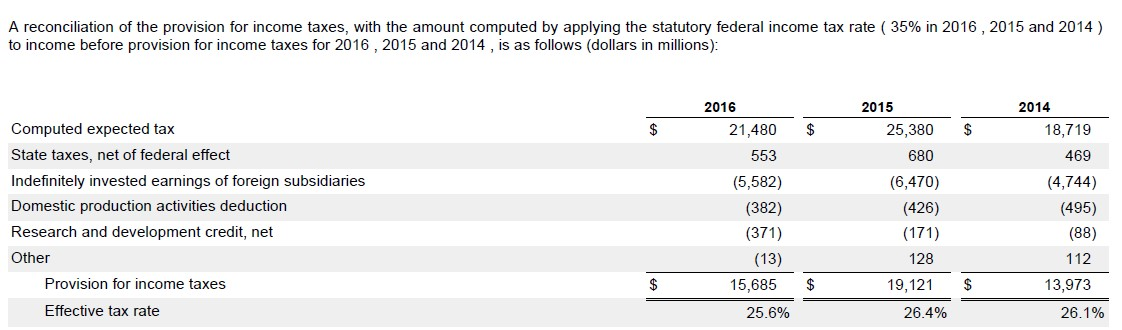As you can see, the effective tax rate is significantly lower because of lower tax rates the company faces outside the United States.

### Tax rate in the WACC calculation

If the current effective tax rate is significantly lower than the statutory tax rate and you believe the tax rate will eventually rise, slowly ramp up the tax rate during the stage-1 period until it hits the statutory rate in the terminal year. If, however, you believe the differences between the effective and marginal taxes will endure, use the lower tax rate.

## Cost of equity

Cost of equity is far more challenging to estimate than cost of debt. In fact, multiple competing models exist for estimating cost of equity: Fama-French, Arbitrary pricing theory (APT) and the Capital Asset Pricing Model (CAPM).

The CAPM, despite suffering from some flaws and being widely criticized in academia, remains the most widely used equity pricing model in practice.

Before diving into the CAPM, let’s first understand why the cost of equity is so challenging to estimate in the first place.

Companies raise equity capital and pay a cost in the form of dilution. Equity investors contribute equity capital with the expectation of getting a return at some point down the road. The riskier future cash flows are expected to be, the higher the returns that will be expected. However, quantifying cost of equity is far trickier than quantifying cost of debt.

That’s because unlike debt, which has a clearly defined cash flow pattern, companies seeking equity do not usually offer a timetable or a specific amount of cash flows the investors can expect to receive.

This creates a major challenge for quantifying cost of equity. At the same time, the importance of accurately quantifying cost of equity has led to significant academic research. There are now multiple competing models for calculating cost of equity.

### The capital asset pricing model (CAPM)

The capital asset pricing model (CAPM) is a framework for quantifying cost of equity. The CAPM divides risk into two components:

• Unsystematic (company-specific) risk: Risk that can be diversified away (so ignore this risk).
• Systematic risk: The company’s sensitivity to market risk can’t be diversified away, so investors will demand returns for assuming this risk.

Since the CAPM essentially ignores any company-specific risk, the calculation for cost of equity is simply tied to the company’s sensitivity to the market. The formula for quantifying this sensitivity is as follows.

### Cost of equity formula

Cost of equity = Risk free rate +[β x ERP]

• β (“beta”) = A company’s sensitivity to systematic risk
• ERP (“Equity risk premium”) = The incremental risk of investing in equities over risk free securities

### The risk-free rate

The risk-free rate should reflect the yield of a default-free government bond of equivalent maturity to the duration of each cash flow being discounted.

The current yield on a U.S. 10-year bond is the preferred proxy for the risk-free rate for U.S. companies. For European companies, the German 10-year is the preferred risk-free rate. The Japan 10-year is preferred for Asian companies.

#### Yields on government bonds (Source: WSJ, 11/6/2017)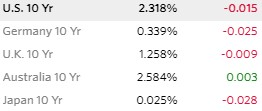How much extra return above the risk-free rate do investors expect for investing in equities in general?

For example, if you plan to invest in the S&P 500 — a proxy for the overall stock market — what kind of return do you expect? Certainly you expect more than the return on U.S. treasuries, otherwise why take the risk of investing in the stock market? This additional expected return that investors expect to achieve by investing broadly in equities is called the equity risk premium (ERP) or the market risk premium (MRP).

But how is that risk quantified? The prevalent approach is to look backward and compare historical spreads between S&P 500 returns and the yield on 10-yr treasuries over the last several decades. The logic being that investors develop their return expectations based on how the stock market has performed in the past.

There are a variety of ways of slicing and dicing past returns to arrive at an ERP, so there isn’t one generally recognized ERP. Below we list the sources for estimating ERPs.

• Morningstar/Ibbotson: Provides a yearbook with various ERPs for a fee
• Duff & Phelps: Provides ERPs for a fee

The ERP usually ranges from 4-6%.

#### Size and country risk premiums

In practice, additional premiums are added to the ERP when analyzing small companies and companies operating in higher-risk countries:

Cost of equity =  risk free rate + SCP + CRP +β x ERP

Where:

• SCP = small company premium
Mid cap (\$800m-4b) 0.5%
Small cap (\$200m-800m) 1.0%
Micro-cap (<\$200m) 2.5%

Source: Ibbotson

United States 0.0%
United Kingdom 0.5%
Germany 0.0%
Australia 0.0%
France 0.5%
China 1.1%
India 3.4%
Middle East 1.4%
Eastern Europe 3.1%
Brazil 3.0%
Africa 5.9%

Source: Damodaran

## Calculating beta

The final calculation in the cost of equity is beta. It is the only company-specific variable in the CAPM.

Beta in the CAPM seeks to quantify a company’s expected sensitivity to market changes. For example, a company with a beta of 1 would expect to see future returns in line with the overall stock market.

Meanwhile, a company with a beta of 2 would expect to see returns rise or fall twice as fast as the market. In other words, if the S&P were to drop by 5%, a company with a beta of 2 would expect to see a 10% drop in its stock price because of its high sensitivity to market fluctuations.

The higher the beta, the higher the cost of equity because the increased risk investors take (via higher sensitivity to market fluctuations) should be compensated via a higher return.

### Calculating raw (historical) beta

How do investors quantify the expected future sensitivity of the company to the overall market? Just as with the estimation of the equity risk premium, the prevailing approach looks to the past to guide expected future sensitivity. For example, if a company has seen historical stock returns in line with the overall stock market, that would make for a beta of 1. You would use this historical beta as your estimate in the WACC formula.

The problem with historical beta is that the correlations between the company’s stock and the overall stock market ends up being pretty weak.

There are several sources for beta:

All of these services calculate beta based on the company’s historical share price sensitivity to the S&P 500, usually by regressing the returns of both over a 60 month period.

The problem with historical beta is that the correlation between the company’s stock and the overall stock market ends up being pretty weak. The reason for this is that in any given period, company specific issues may skew the correlation. For example, while you might expect a luxury goods company’s stock to rise in light of positive economic news that drives the entire stock market up, a company-specific issue (say mismanagement at the company) may skew the correlation.

Thus, relying purely on historical beta to determine your beta can lead to misleading results. Remember, you’re trying to come up with what beta will be.

To address this, Bloomberg, Barra and other services that calculate beta have tried to come up with improvements to arrive at “adjusted beta.” The adjusted beta is essentially a historical beta calculation massaged to get the beta closer to 1. This is only a marginal improvement to the historical beta.

no beta is available for private companies because there are no observable share prices. That’s where the industry beta approach comes in.

Below, we see a Bloomberg screen showing Colgate’s raw and adjusted beta. Bloomberg calculates beta by looking at the last 5 years’ worth of Colgate’s stock returns and compares them to S&P returns for the same period. Using beta as a predictor of Colgate’s future sensitivity to market change, we would expect Colgate’s share price to rise by 0.632% for a 1% increase in the S&P 500.

For the statisticians among you, notice Bloomberg also includes r squared and standard errors for this relationship, which shows you how reliable beta is as a predictor of the future correlation between the S&P and Colgate’s returns. A regression with an r squared of 0.266 is generally considered very uncorrelated (an r squared of 1 is perfect correlation, while 0 is no correlation).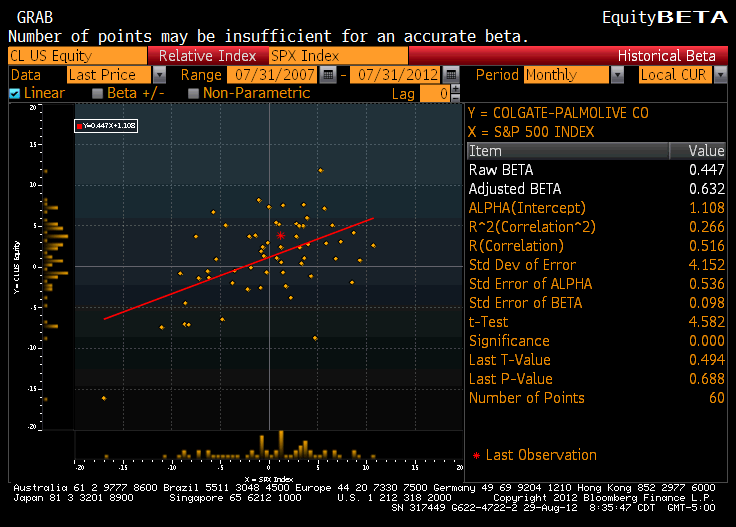## Industry beta

Despite the attempts that beta providers like Barra and Bloomberg have made to try and mitigate the problem outlined above, the usefulness of historical beta as a predictor is still fundamentally limited by the fact that company-specific noise will always be commingled into the beta. Making matters worse is that as a practical matter, no beta is available for private companies because there are no observable share prices. That’s where the industry beta approach comes in.

The industry beta approach looks at the betas of public companies that are comparable to the company being analyzed and applies this peer-group derived beta to the target company. This approach eliminates company-specific noise. It also enables one to arrive at a beta for private companies (and thus value them).

### Unlevered to levered beta formula

The main challenge with the industry beta approach is that we cannot simply average up all the betas. That’s because companies in the peer group will likely have varying rates of leverage. Unfortunately, the amount of leverage (debt) a company has significantly impacts its beta. (The higher the leverage, the higher the beta, all else being equal.) Fortunately, we can remove this distorting effect by unlevering the betas of the peer group and then relevering the unlevered beta at the target company’s leverage ratio. We do this as follows.

### Unlevering peer group beta

For each company in the peer group, find the beta (using Bloomberg or Barra as described in approach #2), and unlever using the debt-to-equity ratio and tax rate specific to each company using the following formula:

β Unlevered = β(Levered) / [1+ (Debt/Equity) (1-T)]

### Relevering beta

Once all the peer group betas have been unlevered, calculate the median unlevered beta and relever this beta using the target company’s specific debt-to-equity ratio and tax rate using the following formula:

β Levered = β(Unlevered) x [1+(Debt/Equity) (1-T)]

This approach will yield a beta that is usually more reliable than the beta gotten from the other approaches we’ve described. In our complete step by step financial modeling training program we build a fully integrated financial model for Apple and then, using a DCF valuation, we estimate Apple’s value. Part of the work involves calculating an industry beta from Apple’s peer — here’s what that looks like: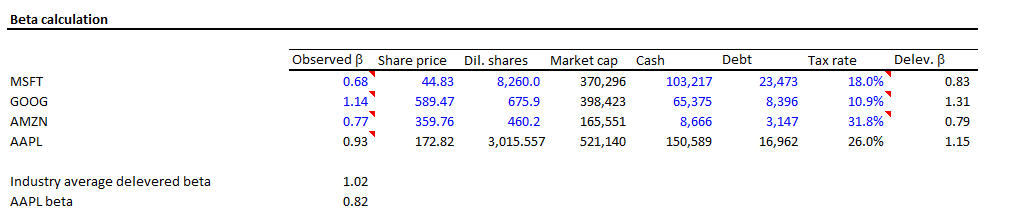Notice how Apple’s observed beta was 0.93 but the relevered industry beta was more than 10% lower: 0.82. Believe it or not, that can have a significant impact on Apple’s valuation (see below).

## WACC in the real world

Weighted Average Cost of Capital (WACC) is a critical assumption in valuation analyses. The assumptions that go into the WACC formula often make a significant impact on the valuation model output. In this guide, we’ve broken down all the components of WACC and addressed many of the nuances that financial analysts must keep in mind. Let’s now take a look at a few screenshots from the model we build in our complete step-by-step financial modeling training program to see exactly how 1) Apple’s WACC is calculated and 2) How the WACC calculation directly impacts Apple’s valuation: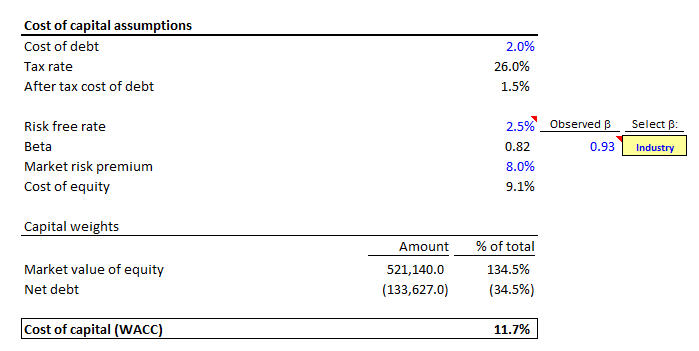According to our estimate, Apple’s WACC is 11.7%. Notice the user can choose from an industry beta approach or the traditional historical beta approach. In addition, notice that in this particular scenario, we are using an 8% equity risk premium assumption. This is very high; Recall we mentioned that 4-6% is a more broadly acceptable range. The impact of this will be to show a lower present value of future cash flows.

Let’s now look at a sensitivity analysis to see how sensitive Apple’s valuation is to changes in the WACC assumptions (as well as the long term growth assumption):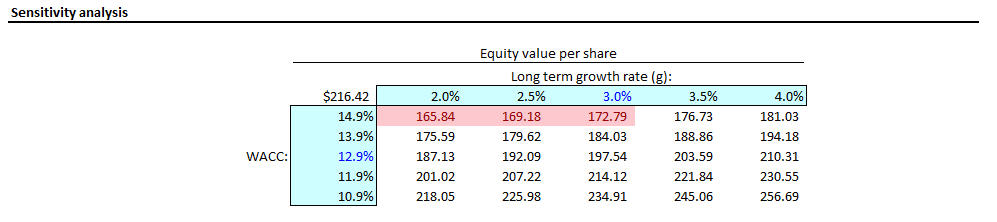1 If interest rates have changed substantially since debt issuance, the market value of debt could have deviated from book values materially. In this case, use the market price of the company’s debt if it is actively traded.Inline FeedbacksSusan Yan
June 2, 2018 11:20 pm

How to compute the total amount of debt in the company’s capital structure that will meet WACCof 10%? GIven: Total equity = \$2,000,000 Before tax of debt is 7% Cost of equity is 16% Corporate income tax rate is 17% I calculate cost of debt is 5.81%, but I do… Read more »Mohd
January 16, 2020 3:11 am

Can you help in this question below, WACC is calculated to me as 12.5892.. Is it correct The management of “BK” company is evaluating an investment project that will give a return of 15%. The project requires 10 million LE as a total investments that will be financed as follows:… Read more »ALEXANDER ARAUZ
February 18, 2022 1:23 pm

For the calculation of the debt, usually in the balance sheet we find long-term debt and short-term debt. Which is correct? to consider Total debt, (short term and long term debt), or to take only long term debt for the WACC calculation?Cory Ireland
December 30, 2021 11:46 am

If a company that I’m analyzing has a large NOL balance, should I used 0% as the tax rate in my WACC calculation?Gorata
November 9, 2021 8:43 pm

YesEugene
September 15, 2021 3:38 pm

Can you please explain in simple words on an intuitive level the painful question, when a company issues debt, then EV does not change if this money does not go to operating activities , but for example, having issued a debt today, then the next day, why is the company… Read more »Francis
August 11, 2021 10:53 pm

if a company generates revenue from multiple countries (e.g. Italy, US and Brazil), which country’s inflation differential should we use?Rodolfo Pena
May 13, 2020 11:37 pm

Hi, I’m very curious to what formula was used to calculate the “delev B” for Apple beta, which is 1.15. I‘m assuming you are using the unlevered formula and if you are could you explain by detail, by plugging in values into the formula, how you got to a beta… Read more »Aakriti Thapa
December 19, 2019 10:08 pm

how to find WACC of a company if a company has \$720 million in common stock outstanding. Its cost of equity is 12%. Moreover, it has \$360 million in 6% coupon rate bonds outstanding. The bond is currently sold at par. There are no taxes in the country in which… Read more »

X

The Wall Street Prep Quicklesson Series

7 Free Financial Modeling Lessons

Get instant access to video lessons taught by experienced investment bankers. Learn financial statement modeling, DCF, M&A, LBO, Comps and Excel shortcuts.